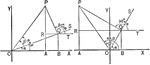### Sum of 2 Acute Angles

Illustration of two triangles, showing the sine of the sum of two acute angles expressed in terms of…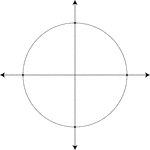### Unit Circle

Illustration of a unit circle (circle with a radius of 1) superimposed on the coordinate plane. The…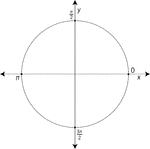### Unit Circle Labeled At Quadrantal Angles

Illustration of a unit circle (circle with a radius of 1) superimposed on the coordinate plane with…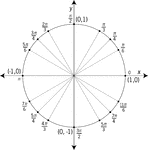### Unit Circle Labeled At Special Angles

Illustration of a unit circle (circle with a radius of 1) superimposed on the coordinate plane with…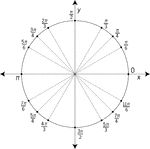### Unit Circle Labeled At Special Angles

Illustration of a unit circle (circle with a radius of 1) superimposed on the coordinate plane with…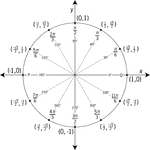### Unit Circle Labeled In 30° Increments With Values

Illustration of a unit circle (circle with a radius of 1) superimposed on the coordinate plane with…### Unit Circle Labeled In 45 ° Increments

Illustration of a unit circle (circle with a radius of 1) superimposed on the coordinate plane with…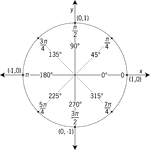### Unit Circle Labeled In 45° Increments With Values

Illustration of a unit circle (circle with a radius of 1) superimposed on the coordinate plane with…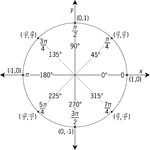### Unit Circle Labeled In 45° Increments With Values

Illustration of a unit circle (circle with a radius of 1) superimposed on the coordinate plane with…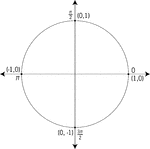### Unit Circle Labeled With Quadrantal Angles And Values

Illustration of a unit circle (circle with a radius of 1) superimposed on the coordinate plane. All…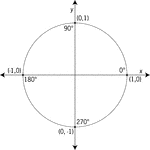### Unit Circle Labeled With Quadrantal Angles And Values

Illustration of a unit circle (circle with a radius of 1) superimposed on the coordinate plane with…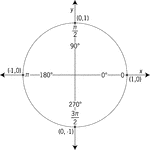### Unit Circle Labeled With Quadrantal Angles And Values

Illustration of a unit circle (circle with a radius of 1) superimposed on the coordinate plane with…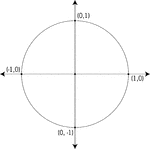### Unit Circle Labeled With Quadrantal Values

Illustration of a unit circle (circle with a radius of 1) superimposed on the coordinate plane. At each…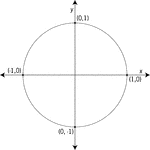### Unit Circle Labeled With Quadrantal Values

Illustration of a unit circle (circle with a radius of 1) superimposed on the coordinate plane with…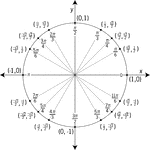### Unit Circle Labeled With Special Angles And Values

Illustration of a unit circle (circle with a radius of 1) superimposed on the coordinate plane with…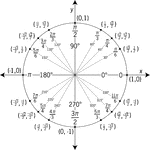### Unit Circle Labeled With Special Angles And Values

Illustration of a unit circle (circle with a radius of 1) superimposed on the coordinate plane with…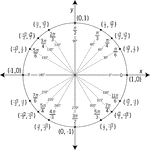### Unit Circle Labeled With Special Angles And Values

Illustration of a unit circle (circle with a radius of 1) superimposed on the coordinate plane with…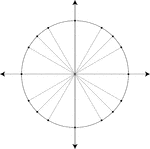### Unit Circle Marked At Special Angles

Illustration of a unit circle (circle with a radius of 1) superimposed on the coordinate plane. All…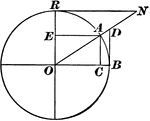### Versedsine and Coversedsine in Circle

The coversedsine is equal to 1 minus the sine. In this figure, AE is parallel to OB; hence, EO = AC…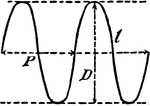### Trigonometric Curve

A curve that is a function and resembles the sine or cosine curve.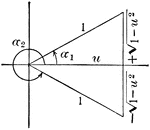### Angle Expressed As An Inverse Function

Illustration that can be used to show that when given an angle, expressed as an inverse function of…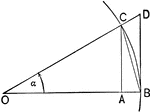### Triangles and Sectors in Quadrant I

Illustration of an angle &alpha with the vertex at the center, O, of a circle with radius OB. AC and…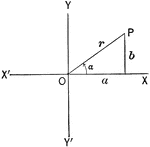Illustration of an angle &alpha with the terminal side used to draw a triangle in quadrant I.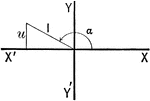Illustration of an angle with the terminal side used to draw a triangle in quadrant II.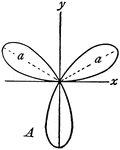### Rose

"In geometry, certain transcendental curves having, in polar coordinates, equations of the form ρ…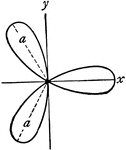### Rose

"In geometry, certain transcendental curves having, in polar coordinates, equations of the form ρ…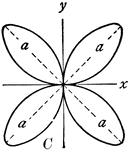### Rose

"In geometry, certain transcendental curves having, in polar coordinates, equations of the form ρ…### Rose

"In geometry, certain transcendental curves having, in polar coordinates, equations of the form ρ…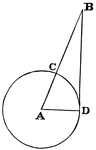### Circle with Secant

A secant is "a line which cuts a figure in any way. Specifically, in trigonometry, a line from the center…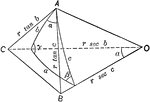### Relationships In A Spherical Triangle

Illustration used, with the law of cosines, to find the relation between the three sides and an angle…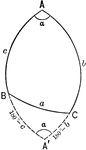### Relationships In A Spherical Triangle

Illustration used to extend the law of cosines when finding the relation between the three sides and…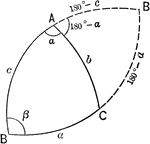### Relationships In A Spherical Triangle

Illustration used to extend the law of cosines when finding the relation between the three sides and…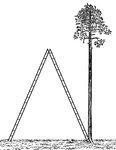Illustration of a giant stepladder, sometimes called a skyscraper stepladder, that is opened next to…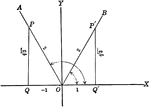### Trigonometric Reference Triangles/Angles (60 degrees) Drawn in Quadrants

Trigonometric reference triangles/angles drawn for 60 degree reference angel in quadrants I and II.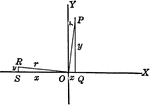### Trigonometric Reference Triangles/Angles Drawn in Quadrants

Trigonometric reference triangles/angles drawn for reference angel in quadrants I and II. This illustration…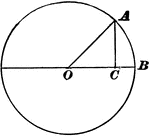### Trigonometry Triangle to Show Sine, Cosine, and Tangent

Right triangle OCA, inside of Circle O is used to show that side AC is "opposite" O and side OC is "adjacent"…